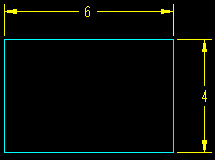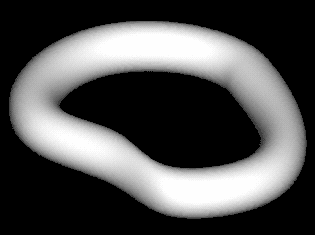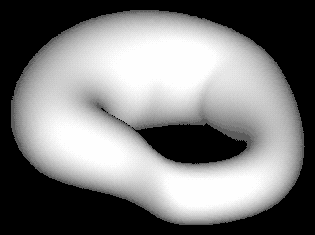>>>  Go to Pro/E Tips Index  >>>
>>>  Page to Previous Pro/E Tip  >>>

October 1999

Using Equations - Part 2

In our September Tip of the Month we began a discussion about using equations in relations.  As promised, we have the answers to the questions, as well as a continuation of our discussion on using equations.

 1. The cross members in the September Figure 2 are a pattern, and the design intent is to define these as being central along the main beams.  Can you write the equations for this? /* MAKE CROSS MEMBERS EQUAL DISTANT FROM ENDS OF I BEAM D8:1=(D4:0-2*D7:1)/(P0:1-1) 2. For the same cross members in the September Figure 2, the length of the protrusion must be controlled by the distance between the main beams.  Can you write the equation? /* MAKE LENGTH FIT DISTANCE BETWEEN BEAMS CROSS_MEMBER_LENGTH:8=D6:1-I_WEB_THICKNESS:0 3. When relations are used in an assembly for component features, where are the relations stored? This was perhaps a trick question -- because you can define it. Select RELATIONS in the ASSEMBLY menu, then in the MODEL REL menu just above he RELATIONS menu, you have the choices for where the relations are stored.  If you choose ASSEM REL, the relations are stored with the Assembly; if you choose PART REL, the relations are stored with the part. TIP:  It is usually a good idea to store the relations with the model which contains the controlled dimensions - in this case, with the part having the clearance holes.  Why? When you modify these dimensions later, it will tell you they are controlled by a relation, and the relation will be right there to look at.  Also, if the part is used without the assembly in session, the dimension will still know it is controlled by a relation. 4. Were you successful with the UDF exercise?  If not, keep trying, and Good luck.

Sketcher Relations:

Sketcher relations are handled much the same as Part or Assembly relations, except that the focus is within the section.  For instance, the relation  sd1=sd0*3/2  will make the one side of the rectangle in Figure AA 50% longer than the other.Can sketcher relations reference dimensions that are not within the sketch?  Yes.  The relation  sd1=d52  is valid, though I would use caution in making relations this way because it can be hard to troubleshoot problems where they are mixed.  It might be better to put this one in the regular part relations.

Now we come to the really fun and powerful part of using sketcher relations - that of using them to control VARIABLE SECTION SWEEPS.  See our June 1999 Tip of the Month for a great example of using section relation equations to drive a sweep.  (Also see November 1998 Tip of the Month for more general information on Variable Section Sweeps.)

Because Variable Section Sweeps evaluate the section all along the trajectory, we get the unique opportunity to allow Pro/E to change the section as it moves along.  We can do that in several ways including, of course, equations.

These equations, if selected correctly, can build shapes that would be difficult or impossible without.  Figures B1 & B2 are a good example.Figure B1 Figure B2

Figure B1 shows the sweep without the equation, Figure B2 shows the sweep after the equation is added.  What equation will set tangency at both ends?

. . .

Think about the functions that are available.

. . .

Here is the equation used:

/* CONTROL BAGEL SHAPE WITH RELATION EQUATION
sd4=20+4*sin(360*trajpar)
/* WHERE sd4 IS THE DIAMETER OF THE SECTION
TIP: A little thought before hand can produce beautiful and complex shapes quite simply.

Good Luck with all your equations.
See you Next Month.

.

SYNTHESIS ENGINEERING SERVICES INC. :  (719) 380-1122